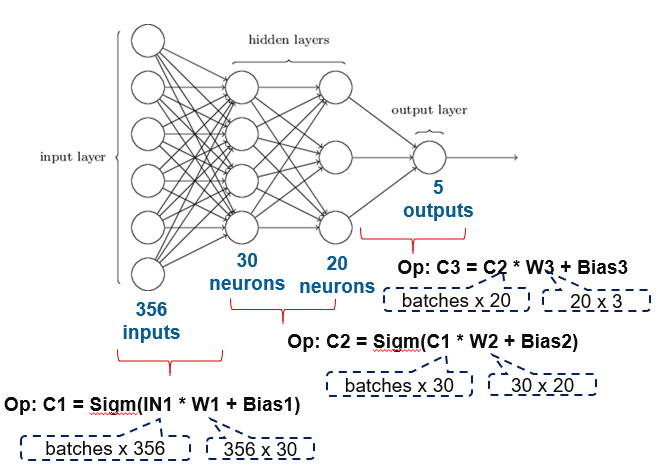# MLP Introduction¶

Multilayer perceptron (MLP) is the general term for a class of feedforward neural networds. The main feature of a MLP is that it consists of at least three layers of nodes: an input layer, a hidden layer and an output layer. A fully connected layer connects all neurons in two adjacent layers together. A fully connected neural network (FCN) addressed by this library is a MLP that only contains fully connected layers. For batched inputs, the basic operations in each fully connected layer include a dense matrix matrix multiplicaiton and an activation function, e.g., sigmoid function.The operations are chainned together to carry out the filtering process addressed by the neural network. Figure 1 illustrates the chainned operations involved in a fully connected neural network. The FCN in Figure 1 consists of 4 layers, namely the input layer, the 2 hidden layers and the output layer. There are 356 inputs, 30 neurons in the first hidden layer, 20 neurons in the second hidden layer and 5 outputs. The activation funtion in each layer is sigmoid function, and the inputs are batched. The implementation of this neural network involves three chainned operations, meaning the results of the previous operation will become the inputs of the next operation. The firt operation C1 = Sigm(IN1 * W1 + Bias1) happens when data are filtered through from the input layer to the first hidden layer. The second operation C2 = Sigm(C1*W2 + Bias2) happens between the first hidden layer and the second hidden layer. The last operation C3 = Sigm(C2 * W3 + Bias3) happens between the second hidden layer and the output layer. Here Sigm denotes the sigmoid function. The input matrix IN1 is formed by batching input vectors. The batch size is denoted by batches. The weight matrices in each layer are denoted by W1, W2 and W3. The bias matrices are denoted by Bias1, Bias2 and Bias3. The results matries are used as input matrices, e.g., C1 and C2. This forms an operation chain.Figure 1. FCN example

The basic primitives in this release are implemented in class Fcn. The activation function primitives provided by this release include relu, sigmoid and tansig.